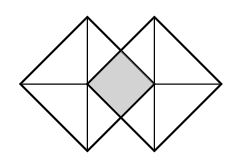### Home > CCA2 > Chapter 3 > Lesson 3.2.5 > Problem3-125

3-125.

Two congruent overlapping squares are shown at right. If a point inside the figure is chosen at random, what is the probability that it will not be in the shaded region?

Draw the figure on your graph paper to scale. Divide the shape into equal parts. Count how many are not shaded.

$\frac {6}{7}$